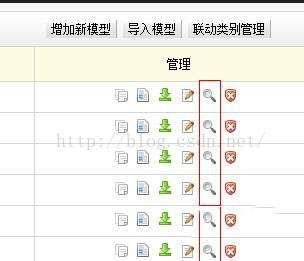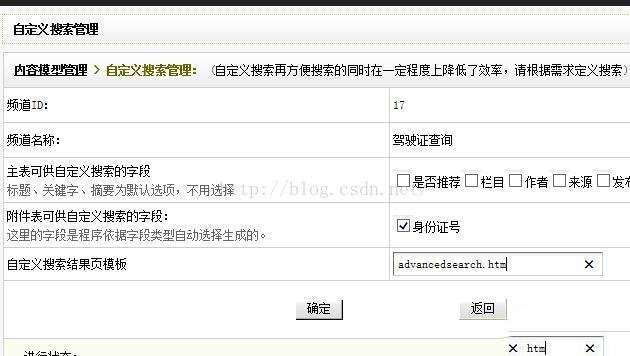# 织梦dedecms二次开发个身份证驾驶证查询功能

##### 92建站   2021-05-10   收藏本文(此图片来源于网络，如有侵权，请联系删除! )(此图片来源于网络，如有侵权，请联系删除! )

if(isset(\${\$var}) && trim(\${\$var})!='')

{

\${\$var} = stripslashes(\${\$var});

\${\$var} = preg_replace("#[\|\"\r\n\t%\*\?\(\)\\$;,'%<>]#", "", trim(\${\$var}));

//\$where .= " AND addon.\$var LIKE '%\${\$var}%'";

\$where .= " AND addon.\$var = '\${\$var}'";

}

else{

ShowMsg('身份证不能为空,或输入不正确',-1,0);exit;}

else

{

\$sql = urldecode(\$sql);

\$query = \$sql;

}

else

{

\$sql = urldecode(\$sql);

\$query = \$sql;

}

//print_r(\$sql);exit;

\$searchinfo2 = \$dsql->GetOne(\$sql);

if(!is_array(\$searchinfo2))

{

showmsg('查询的信息不存在,请检查输入','-1');

exit();

}